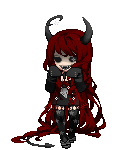## *~*~ CCONX ~*~*Registered: 06/06/2010

Gender: Male

Birthday: 06/18

Personal Website

## *~*~ My Store ~*~*

*=v=^=v=^=v=^=v=^=v=^=v=^=v=^=v=^=v=^=v=^=v=^=v=^=v=^=v=*

Welcome to my store!

All my items are for sale for a week so get them while you can!

I guarantee the cheapest price, and if you see a price cheaper then mine, tell me and Ill make sure you get it cheaper!

And, become a loyal enough costumer, and Ill even be willing to make special deals with negociable prices!

*=^=v=^=v=^=v=^=v=^=v=^=v=^=v=^=v=^=v=^=v=^=v=^=v=^=v=^=*

View Store

## My Lovely Stalkers

MaliciousLust Azira on 06/19/2020
RoyaI Saint on 04/15/2020## *~*~ Some s**t To Read ~*~*

*=v=^=v=^=v=^=v=^=v=^=v=^=v=^=v=^=v=^=v=^=v=^=v=^=v=^=v=*

...Love me...

...Hate me...

...Leave me to die...

*=v=^=v=^=v=^=v=^=v=^=v=^=v=^=v=^=v=^=v=^=v=^=v=^=v=^=v=*

Name: Charles

Gender: Male (dress avi as a female sometimes though)

Age: 21

DOB: June 18th 1996

Relationship Status: Forever alone

Likes: Anime, music, drawing, art, animals, new friends

Dislikes: Unfair hatred, eggplant

I'm a furry.
I'm an otaku.
I'm a weeb.
I'm an introvert.
I'm an artist.
I'm a writer.
I love music.
I sing (I'm sure the shower enjoys it).
Huge Homestuck fan.
Massive Undertale fan.
Space Station 13 nerd.

Anything else you wanna know just ask.

*=v=^=v=^=v=^=v=^=v=^=v=^=v=^=v=^=v=^=v=^=v=^=v=^=v=^=v=*

Places to find me:

I'm not on much, so here are places you can find me other than here;

Skype - xalexinfernox / CCONX

Kik - CCONX

Discord - CCONX #5078

Snapchat - CCONX

Telegram - CCONX

BYOND - CCONX

(Remember; When you friend me always send a message letting me know you got my information from here, and more importantly, who you are.)

*=^=v=^=v=^=v=^=v=^=v=^=v=^=v=^=v=^=v=^=v=^=v=^=v=^=v=^=*

For art by me,
Go to my devintArt profile CCONX

*=^=v=^=v=^=v=^=v=^=v=^=v=^=v=^=v=^=v=^=v=^=v=^=v=^=v=^=*## *~*~ People I Kind Of Know ~*~*

View All Friends

Report | 06/22/2017 12:18 pmhow you been?
Report | 04/02/2017 8:00 am*~*~*~*~*~*~*~*~*~*~*~*~*~*~*~*~*~*
I'm doing good yum_strawberry

*~*~*~*~*~*~*~*~*~*~*~*~*~*~*~*~*~*
Report | 03/19/2017 6:43 amyum_puddi How have you been? yum_puddi
Report | 02/05/2017 9:41 amemotion_bigheart emotion_bigheart emotion_bigheart MISS YOU!
Report | 01/08/2017 7:20 pmheart love!!!!! heart
Report | 01/03/2017 6:10 pmReport | 11/11/2016 2:40 pmCCONX!!! >^o^<
Report | 05/18/2016 4:31 pmlol I can tell
Report | 05/09/2016 2:26 pmwhere chu at??
Report | 03/17/2016 8:21 pmJust stopping by to say,
I dig your profile music and background.

So have a nice day~